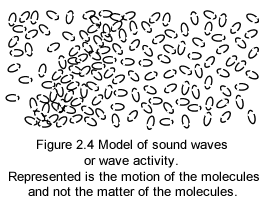Confined activity (temperature) mathematical equivalent (§2.3)
Using physics' conventional quantities, units and mathematical operations, one can show that our concept of temperature denoted here Θ (read theta) is equivalent to a speed squared: Θ = v2.
In the following, English's decimal comma and point are in use. They coincide in that order respectively to French's decimal point and comma! 4.184 in this page is written 4,184 in France. And 4,184 in this page would be written 4.184 in France.
The Calorie, an old unit of energy, has been redefined as being equivalent to 4.2 Joules. 1 calorie (thermo chemical) = 4.184 joule exactly.
4.184 joule = 1 calorie(1)
The Joule also a unit of energy, itself equivalent to a force multiplied by distance, is defined as 1 Joule = 1 Newton x 1 meter.
4.184 x 1 Newton x 1 meter = 1 calorie(2)
The Newton a unit of force, itself equivalent to the acceleration of matter, is defined as 1 Newton = 1 kilogram of matter accelerated at the rate of 1 meter per second second.
4.184 x 1 Kg x (1 meter/ 1 sec sec) x 1 meter = 1 calorie(3)
But the calorie a unit of heat (heat being a specific form of energy), is defined as being the heat added to one gram of water to raise it from 14.5 to 15,5 degree Celsius.
4.184 x 1 Kg x (1 meter/ 1 sec sec) x 1 meter = 1o Celsius x 1 gram(4)
or:
4.184 x 1000 gram x (1 meter/ 1 sec sec) x 1 meter = 1o Celsius x 1 gram(5)
which can be rewritten:
4,184 gram x (1 meter/ 1 sec sec) x 1 meter = 1o Celsius x 1 gram(6)
Dividing each side by one gram, and multiplying each side by the factor sec x sec:
4,184 x 1 meter x 1 meter = 1o Celsius x 1 sec sec(7)
or:
(1 meter)2 / (1 sec)2 = 1o Celsius / 4,184(8)
Or within a constant :
Θo = v2(9)
A temperature can be represented as a speed squared, or maybe as the product of 2 speeds, as Maxwell density graph suggests.
Model of sound; wave-activity (§2.4)Sound waves ride the confined-activity of air molecules; as such confined-activity is the subjacent-motion of sound waves; should the air molecules be frozen stiff there would be no sound.
All waves in gravimotion are illustrated as a dynamic modification of a background motion-activity of type Maxwellian.
Note that, in this sound waves specific case, it also means that the molecules themselves or their matter is not part of the model.
When a sound wave goes by, the air confined-activity is modified into dynamic heaps and troughs of MOs.
In the illustration the peaks and the troughs of the wave, which are respectively represented as agglomerations and depletions of motion-occurrences, are moving from left to right.